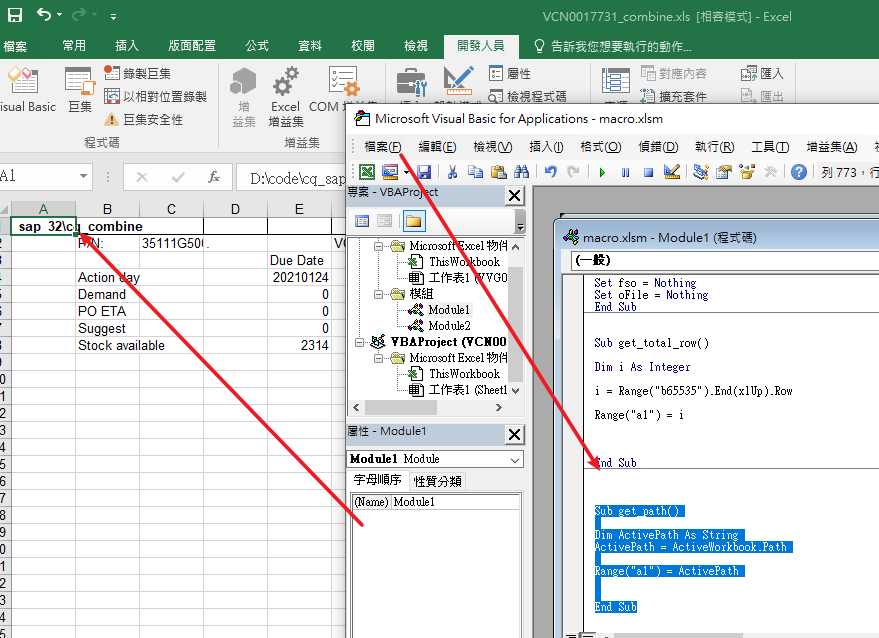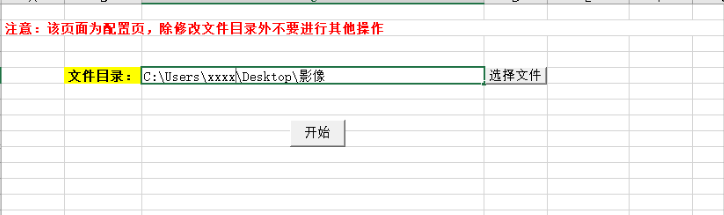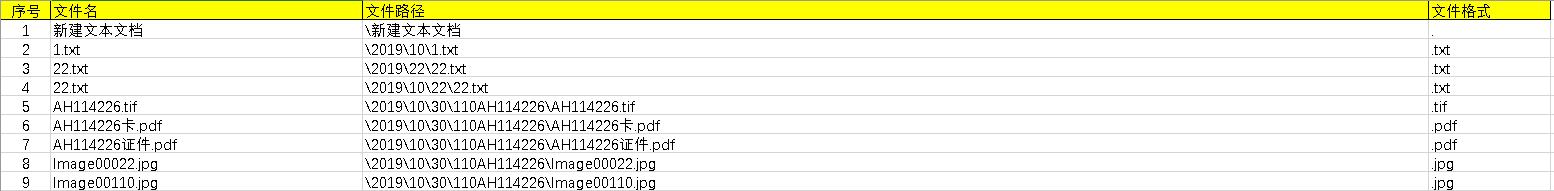• 1 2 3 4 5 Sub?s() ????Dim?pth$????pth?=?ThisWorkbook.Path ????MsgBox?"本文件的路径为："?&?pth End?Sub  1 2 3 4 5 Sub? s() ???? Dim? pth$

????
pth?=?ThisWorkbook.Path

????
MsgBox?
"本文件的路径为："?
&?pth

End?
Sub


展开全文java python linux
• Sub get_path() Dim ActivePath As String ActivePath = ActiveWorkbook.Path Range("a1") = ActivePath End Sub 参考 看67433的回答 https://zhidao.baidu.com/question/2117151906662330347.html
Sub get_path()

Dim ActivePath As String
ActivePath = ActiveWorkbook.Path

Range("a1") = ActivePath

End Sub参考
看67433的回答 https://zhidao.baidu.com/question/2117151906662330347.html
展开全文• 返回应用程序完整路径  Application.Path  ...返回当前默认文件路径：  Application.DefaultFilePath  Application.ActiveWorkbook.Path 只返回路径  Application.ActiveWorkbook.FullName
返回应用程序完整路径  Application.Path   返回当前工作薄的路径  ThisWorkbook.Path   返回当前默认文件路径：  Application.DefaultFilePath

Application.ActiveWorkbook.Path   只返回路径  Application.ActiveWorkbook.FullName   返回路径及工作簿文件名  Application.ActiveWorkbook.Name   返回工作簿文件名

展开全文• 开始参考了 VBA获取某文件夹下所有文件和子文件目录的文件中的代码，按照此方式获取的结果有问题。 问题1 无法获取目录名中包含“.”的子目录 '-- 获得所有子目录 Do Until i > k f = Dir(file(i), vbDirectory)...
公司运营部门需要把影像资料梳理一遍，文件目录特别多，文件量也大，大概40多个G。自己写了一个读取目录下所有子文件的脚本 开始参考了 VBA获取某文件夹下所有文件和子文件目录的文件中的代码，按照此方式获取的结果有问题。 问题1 无法获取目录名中包含“.”的子目录
'-- 获得所有子目录
Do Until i > k
f = Dir(file(i), vbDirectory)
Do Until f = ""
If InStr(f, ".") = 0 Then
k = k + 1
ReDim Preserve file(1 To k)
file(k) = file(i) & f & "\"
End If
f = Dir
Loop
i = i + 1
Loop

代码中使用InStr(f, “.”) = 0 判断，只要名字中包含"."就按照文件处理
问题2 无法获取扩展名为空的文件
'-- 获得所有子目录下的所有文件
For i = 1 To k
f = Dir(file(i) & "*.*")    '通配符*.*表示所有文件，*.xlsx Excel文件
Do Until f = ""
'Range("a" & x) = f
x = x + 1
f = Dir
Loop
Next

于是，自己实现了一个支持文件夹名称带“.”或文件名不带扩展名的。 实现过程 新建一个文件，在sheet1中增加两个按钮，一个用来选取文件夹，一个用来执行查询
选择文件脚本
Option Explicit
Sub 打开文件夹()

With Application.FileDialog(msoFileDialogFolderPicker)
If .Show = -1 Then
Worksheets("Sheet1").Range("C5").Value = .SelectedItems(1)
End If
End With

End Sub


执行脚本

Sub 按钮1_Click()

On Error Resume Next

Dim folderObj As Object
Dim currFolder
Dim fdCnt As Integer

Dim sDir As String

Dim dirExist, f As String
Dim file(), subFolder(), allfd() As String
Dim fileNum, k, x, idx, i, j, listNum
Dim threeDir As String

fileNum = 1
x = 1
k = 1
j = 0
i = 1

sDir = Worksheets("Sheet1").Range("C5").Value

'=== 0.清除数据=============================================
Sheet2.UsedRange.Clear

Worksheets("Sheet2").Range("A1").Value = "序号"
Worksheets("Sheet2").Range("C1").Value = "文件名"
Worksheets("Sheet2").Range("D1").Value = "文件路径"
Worksheets("Sheet2").Range("E1").Value = "文件格式"
Worksheets("Sheet2").Range("E1").Interior.Color = RGB(255, 255, 0)
Worksheets("Sheet2").Range("A1").Interior.Color = RGB(255, 255, 0)
Worksheets("Sheet2").Range("C1").Interior.Color = RGB(255, 255, 0)
Worksheets("Sheet2").Range("D1").Interior.Color = RGB(255, 255, 0)
Worksheets("Sheet2").Range("E1").Borders.LineStyle = xlContinuous
Worksheets("Sheet2").Range("A1").Borders.LineStyle = xlContinuous
Worksheets("Sheet2").Range("C1").Borders.LineStyle = xlContinuous
Worksheets("Sheet2").Range("D1").Borders.LineStyle = xlContinuous

'=== 1.判断选择的文件夹是否有效===============================

dirExist = dir(sDir, vbDirectory)
If dirExist = "" Then
MsgBox ("选择的文件夹无效")
Exit Sub
End If

'=== 2.获取所有子目录======================================

ReDim subFolder(1 To i)

subFolder(1) = sDir & "\"
f = dir(subFolder(1), vbDirectory)
Do Until f = ""
If f <> "." And f <> ".." Then
If (GetAttr(subFolder(1) & f) And vbDirectory) = 16 Then
'Worksheets("Sheet3").Range("A" & k).Value = subFolder(1) & f & "\"
k = k + 1
ReDim Preserve subFolder(1 To k)
subFolder(k) = subFolder(1) & f & "\"
End If
End If
f = dir
Loop
i = i + 1

Dim tmp As Integer
tmp = 0

For Each fd In subFolder
tmp = tmp + 1
ReDim Preserve allfd(1 To tmp)
i = 1
k = 1
Erase file
ReDim file(1 To i)
file(i) = fd
allfd(tmp) = fd
Worksheets("Sheet3").Range("B" & tmp).Value = allfd(tmp)

If subFolder(1) = file(i) Then
f = dir
i = i + 1
Else

Do Until i > k
f = dir(file(i), vbDirectory)
Do Until f = ""
If f <> "." And f <> ".." Then
If (GetAttr(file(i) & f) And vbDirectory) = 16 Then
k = k + 1
ReDim Preserve file(1 To k)
file(k) = file(i) & f & "\"
tmp = tmp + 1
ReDim Preserve allfd(1 To tmp)
allfd(tmp) = file(i) & f & "\"
' Worksheets("Sheet3").Range("B" & tmp).Value = allfd(tmp)
End If
End If
f = dir
Loop
i = i + 1
Loop
End If
Next

'=== 3.获取所有子目录下的文件======================================
'
Dim threeStr As String

x = 2
idx = 1
For i = 1 To tmp

f = dir(allfd(i) & "*.*")
Do Until f = ""
Worksheets("Sheet2").Range("A" & x).Value = idx
Worksheets("Sheet2").Range("C" & x).Value = f
Worksheets("Sheet2").Range("D" & x).Value = Replace(allfd(i), sDir, "") & f
'Worksheets("Sheet2").Range("E" & x).Value = getFileType(f)

'Worksheets("Sheet2").Range("B" & x).NumberFormatLocal = "@"
'Worksheets("Sheet2").Range("B" & x).Value = getToubaodanHao(sDir, allfd(i))

f = dir
x = x + 1
idx = idx + 1
Loop

Next

End Sub


最终效果：参考
W3CSchool VBA教程VBA获取某文件夹下所有文件和子文件目录的文件VBA 快速入门
展开全文• &lt;strong&gt;VBA获取某文件夹下所有文件，或子文件目录的文件&lt;/strong&gt; '-----------------------------------excel 子目录 目录
• Dim wb As Workbook paths = ThisWorkbook.Path &amp;amp; &quot;\&quot; Set wb = Workbooks.Open(paths &amp;amp; &quot;test.xlsx&quot;) wb.Close
• Sub GetFiles() Application.... Set ff = fso.getfolder(ThisWorkbook.Path) 'ThisWorkbook.Path是当前代码文件所在路径，路径名可以根据需求修改 ActiveSheet.UsedRange.ClearContents a = 2 Dim...
• 遍历指定文件夹，根据文件夹内文件内容，取得文件信息，并汇总在一个EXCEL中
• Sub main() '选择采集文件获取采集的文件路径 FilePath = SelectFile call CopyAgilent(FilePath) End Sub '选择ncsCS采集文件 Function SelectFile() With Application.FileDialog(msoFileDialogFilePicker) ....excel 经验分享
• 获取当前文件夹的名称(CurDir 函数)当你使用文件时，经常会需要知道当前文件夹的名称，你使用CurDir函数轻易地获取该信息：CurDir([drive])Drive是一可选参数，如果你忽略它，VBA将使用当前驱动(drive)。CurDir函数...
• VBA个人 最初的想法是为personal.xlsb一个存储库。...在我看来，构建一个样板文件（作为每个VBA项目的起点）将是很长的。 在2016年的某个地方，我决定将我正在使用的所有有用的VBA代码放在一个存储库中。 该存储库当前
• VBA读取文件夹下所有文件的三种方法，能够读取到子文件夹下所有的文件
• 从本地html文件中提取内容,支持弹框显示选择的HTML页面，获取对应的标签，或者对应的文本，修改下就可以用了
• 01、获取打开文件的工作路径和工作名 '====================================================================== '功能： 获取打开文件的工作路径和工作名 '函数名： selectExcelfile '参数1： ThisDirPath 需查找...
• iamlaosong文 文件的日期时间分创建时间和修改时间，文件一旦创建，创建...1、取文件的修改时间VBA有专门的函数FileDateTime，如下面的语句： PicName = ThisWorkbook.Path &amp; "\YiCode.bmp" ...
• 经常用到excel，以下是遍历当前文件夹下的excel表。 Sub bl() Dim myPath As String, myFile As String Dim i As Integer Dim Arr() As String myPath = ThisWorkbook.Path &amp;amp;amp; &amp;...
• 文章目录 读取当前文件名 读取当前文件名 Version = Left(ThisWorkbook.Name, InStr(ThisWorkbook.Name, ".") - 1) '读取的文件名除去后缀 FileKey = Version & ".xlsm" '读取的文件名加上后缀 参考地址：...excel
• '读取当前文件夹下txt文件的文件名,写入sheet1的第一列 Dim r As Long Dim Fso As Object Dim myfile As Object Dim mySheet As Worksheet Dim txtFolder As Object r = 1 Set txtFolder = CreateObject("S...excel
• 如果你的信息是Excel文件，那么下面介绍的一招，轻松解决你的困惑 效果图 正常Excel打开状态 从上面的效果图可以看到，其它用户打开的时候，Excel不会显示工作表信息，会觉得是不是这个 Excel ...Excel 用户名称
• 扩展名也可以是其它的xlsx之类的，自己修改扩展名就行了 Sub SaveAllAndCloseXls() Dim FileName As String Dim extensionName As ... FileName = wbk.Name '获取文件的名字，带扩展名的那种，长这样：report.x...
• 大家好，我们今日讲解“VBA信息获取与处理”教程中第十七个专题“文件及文件夹信息的获取及操作”的第一节“判断文件及文件夹是否存在”，这个专题是非常实用的知识点，希望大家能掌握利用。教程会提供配套的程序...
• oleobject o_WorkBook long ll_Row,ll_RowCount,ll_NewRow String ls_SheetName o_WorkBook = Message.PowerObjectParm ll_RowCount = o_WorkBook.Worksheets.Count FOR ll_Row = 1 TO ll_RowCount ...
• 要求：将文件夹1-1.xlsx、文件夹1-1.xlsx移动到文件夹1，依次类推代码及说明：Sub 移动文件()Dim MyFold As Object, MyFile As ObjectDim ipath As String, TargetFolder As String'获取当前文件夹的路径ipath = ...
• Private strFiles(20) ...Private Sub GetFiles(ByVal strFolder As String, ByVal ty As String) ' 获取文件列表 If iFiles = 0 Then Erase strFiles End If If iFiles > 20 Then Exit S...
• 大家好，我们今日讲解"VBA信息获取与处理"教程中第十七个专题"文件及文件夹信息的获取及操作"的第三节"利用VBA获取文件的信息和属性"，这个专题是非常实用的知识点，希望大家能掌握利用。教程会提供配套的程序文件。...
• 该Excel VBA工具可以列出指定目录下所有子目录和文件， 还可以过滤不要的目录，文件，指定匹配的目录和文件...